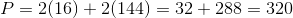# ISEE Lower Level Math : How to find the perimeter of a parallelogram

## Example Questions

### Example Question #20 : Geometry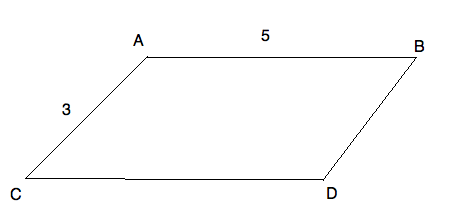What is the perimeter of parallelogram ABCD?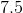Cannot be determinedExplanation:

The perimeter of a parallelogram is very easy to find. You just need to add up all the sides. However, you need to notice that the sides "across" from each other are equal on parallelograms. So, your figure could be redrawn: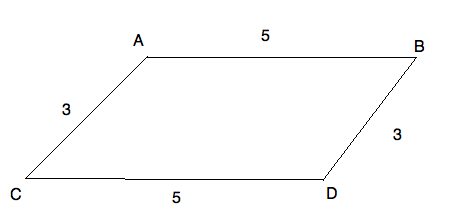The perimeter of your figure is therefore: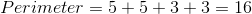### Example Question #1 : Plane Geometry

A parallelogram has a side length of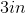.  It also has a side length of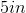.  Calculate the perimeter.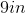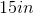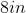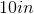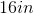Explanation:

A parallelogram has four sides and its opposite sides are equal in length.  Therefore, if it has one side length of, it also has another side length of of.  Since we know one of its side lenghts is, then the remaining side is.  We can add all 4 side lengths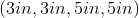to calculate the perimeter.

### Example Question #2 : Plane Geometry

What is the perimeter of a parallelogram if the base is, the other side is, and the height is?Explanation:

The perimeter of a parallelogram is the sum of all four sides or the sum of two times each side length.  The side lengths areandso the perimeter is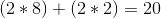.

### Example Question #3 : Plane Geometry

Find the perimeter of the given parallelogram: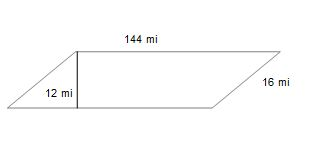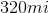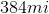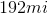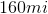Explanation:

Find the perimeter of the given parallelogram:The perimeter of any shape can be found by adding up the lentghs of its sides.

In this case, we have four sides. 2 that are 16 miles long, and 2 that are 144 miles long.

Find perimeter as follows: